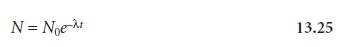Home | | Modern Analytical Chemistry | Radiochemical Methods of Analysis: Theory and Practice

# Radiochemical Methods of Analysis: Theory and Practice

The rate of decay, or activity, for a radioactive isotope follows first-order kinetics

Theory and Practice

The rate of decay, or activity, for a radioactive isotope follows first-order kineticswhere A is the activity, N is the number of radioactive atoms present in the sample at time t, and λ is the radioisotope’s decay constant. Activity is given in units of dis- integrations per unit time, which is equivalent to the number of atoms undergoing radioactive decay per unit time.

As with any first-order process, equation 13.24 can be expressed in an inte- grated form.Substituting equation 13.25 into equation 13.24 givesBy measuring the activity at time t, therefore, we can determine the initial activity, A0, or the number of radioactive atoms originally present in the sample, N0.

An important characteristic property of a radioactive isotope is its half-life, t1/2, which is the amount of time required for half of the radioactive atoms to disinte- grate. For first-order kinetics the half-life is independent of concentration and is given asSince the half-life is independent of the number of radioactive atoms, it remains constant throughout the decay process. Thus, 50% of the radioactive atoms disinte- grate in one half-life, 75% in two half-lives, and 87.5% in three half-lives.

Kinetic information about radioactive isotopes is usually given in terms of the half-life because it provides a more intuitive sense of the isotope’s stability. Know- ing, for example, that the decay constant for 9030Sr is 0.0247 yr–1 does not give an im- mediate sense of how fast it disintegrates. On the other hand, knowing that the half- life for 9030Sr is 28.1 years makes it clear that the concentration of  9030Sr in a sample remains essentially constant over a short period of time.

Study Material, Lecturing Notes, Assignment, Reference, Wiki description explanation, brief detail
Modern Analytical Chemistry: Kinetic Methods of Analysis : Radiochemical Methods of Analysis: Theory and Practice |